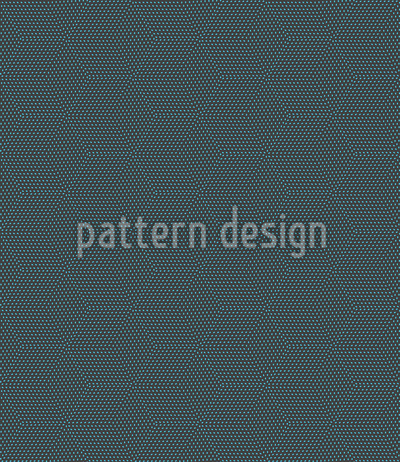••••# Cube Dimension Seamless Vector Pattern Design

Keywords
Line, Confusing, Dimension, 3d, Octagon, Iridescent, Geometric, Cube, Geometry, Stroke, Hexagon, Three-dimensional, Geometric Pattern, Geometrical Cube Dimension design, Cube Dimension vector, Cube Dimension pattern, Cube Dimension all over print, Cube Dimension illustration, Cube Dimension ornament, Cube Dimension pattern design, Cube Dimension artwork, Cube Dimension vector art, Cube Dimension background pattern, Cube Dimension textile, Cube Dimension seamless, Cube Dimension repeating pattern, Cube Dimension surface
Designer Anna Kotlyar ID #8398
Description Great! Confusing design with cube elements consisting of small strokes.
Prices